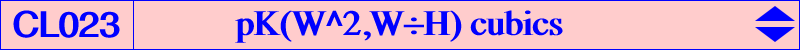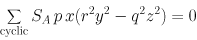Let W = (p : q : r) be a pole of isoconjugation and P* the W-isoconjugate of point P.

The cevian triangle of P* and ABC are orthologic if and only if P lies on pK(W^2,W÷H) where W^2 is the barycentric square of W and W÷H = U is the barycentric quotient of W and H i.e. W^2 = (p^2 : q^2 : r^2) and U = (p SA : q SB : r SC).

Any such cubic is the transform of the Lucas cubic K007 in the isoconjugation with pole W.

pK(W^2,W÷H) contains W and its three harmonic associates and its equation is :Naturally, the Lucas cubic K007 is the simplest example of such cubic with W = G, W^2 = G, U = X(69).

When W = K, we obtain K172 with W^2 = X(32) and U = O.

The following table gives a selection of pK(W^2,U) with centers denoted i for X(i). P refers to Clark Kimberling's Z(U,P) cubics.

 W W^2 U P cubic centers on the cubic 1 6 63 1 K343 1, 9, 19, 40, 57, 63, 84, 610, 1712, 2184 2 2 69 31 K007 2, 4, 7, 8, 20, 69, 189, 253, 329, 1032, 1034 4 393 2 255 2, 4, 278, 281, 393, 1249 6 32 3 75 K172 3, 6, 25, 55, 56, 64, 154, 198, 1033, 1035, 1436 7 279 348 1253 2, 7, 278, 279, 347, 348, 1440 8 346 345 1106 2, 8, 280, 281, 345, 346 9 220 78 269 10 594 306 849 10, 226, 306, 1826, 2321 19 2207 1 326 1, 19, 33, 34, 204, 207, 1096, 2331 31 1501 48 561 37 1500 72 757 57 1407 77 200 63 394 326 1096 1, 63, 77, 78, 326 75 76 304 560 75, 85, 92, 304, 309, 312, 322 100 1252 1332 244 513 1015 905 765 523 115 525 1101